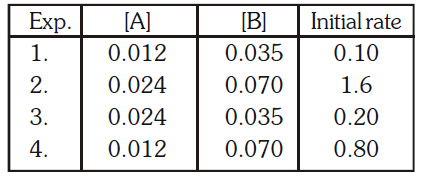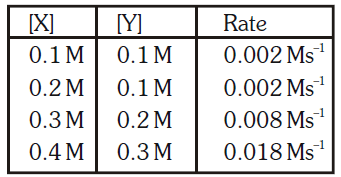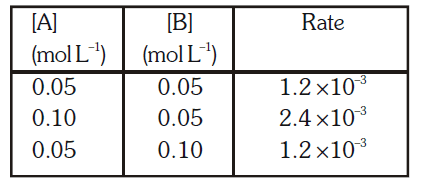Please click on square box [ ] at right top corner for Full Screen

0%
2

All The Best

Created on Byaajkatopper

Chemistry

CHEMICAL KINETICS TEST - 1

1 / 20

Which of the following statement is not correct for the reaction: 4A + B → 2C + 2D
निम्न में से कौनसा कथन अभिक्रिया के लिए सही नही है: 4A+ B→ 2C+ 2D

2 / 20

For a general chemical change 2A+3B → products, the rate of disappearance of A is r1 and of B is r2. The rates r1 and r2 are related as :
एक सामान्य रासायनिक परिवर्तन 2A+3B → उत्पाद के लिए A के विलुप्त होने की दर r1 तथा B कीr2 है। r1 और r2 सम्बन्धित है :

3 / 20

For the reaction H2(g) + Br2(g) → 2HBr(g), the experimental data suggests, Rate = K [H2] [Br2]½. The order for this reaction is 
अभिक्रिया H2(g) + Br2(g) → 2HBr(g) के लिए प्रायोगिक मानों के अनुसार दर = K [H2] [Br2]½ है। इस अभिक्रिया के लिए कोटि है -

4 / 20

Consider the chemical reaction : N2(g) + 3H2(g) → 2NH3(g) The rate of this reaction can be expressed in terms of concentration of N2(g), H2(g) or NH3(g). Identify the correct relationship amongst the rate expressions.
अभिक्रिया N2(g) + 3H2(g) → 2NH3(g) पर विचार कीजिए। इस अभिक्रिया की दर को N2(g), H2(g) या NH3(g) की सान्द्रता के पदों में व्यक्त किया जा सकता है। दर व्यंजकों के मध्य सही सम्बन्ध बताइये :

5 / 20

Select the rate law that corresponds to the data shown for the following reaction A + B → C
अभिक्रिया A+ B → C के लिए निम्नलिखित मानों के अनुसार दर नियम चुनिए6 / 20

Select the law that corresponds to data shown for the following reaction 2A + B → C + D :-
निम्न अभिक्रिया 2A + B → C + D के लिए दिए गए मानों के संगत दर नियम चुनिए7 / 20

The rate law for the single step reaction 2A + B → 2C, is given by 
एक पदीय अभिक्रिया 2A+ B →2C के लिए दर नियम दिया जाता है

8 / 20

For a chemical reaction A + B → product, the order is one with respect to each A and B. Value of x and y from the given data is :
एक रासायनिक अभिक्रिया A+ B→ उत्पाद के लिए A और B के सापेक्ष कोटि एक है। दिए गए मानों से x और y का मान y है9 / 20

In a certain gaseous reaction between X and Y, X + 3Y → XY3 The initial rates are reported as follows The rate law is 
X और Y के मध्य एक निश्चित गैसीय अभिक्रिया X + 3Y → XY3 में प्रारम्भिक दर निम्न प्रकार पायी गई दर नियम है10 / 20

For a hypothetical reaction ; A + B → C the following data were obtained in three different experiments :The rate law will be :
एक काल्पनिक अभिक्रिया A+ B → C के लिए निम्न तीन प्रायोगिक मान प्राप्त होते हैं : दर नियम होगा11 / 20

Point out incorrect statement.
गलत कथन इंगित कीजिए

12 / 20

For the reaction A + B → products, it is found that the order of A is 1 and the order of B is . When the concentration of both A and B are increased four times, the rate will increase by a factor of :
अभिक्रिया A+ B → उत्पाद के लिए यह पाया गया कि A तथा B के सापेक्ष कोटि 1 तथा है। A तथा B की सान्द्रता को चार गुना कर दिया जाए तो अभिक्रिया की दर कितने गुना बढ़ जाएगी :

13 / 20

Calculate the order of the reaction w.r.t. A and B:
A और B के सापेक्ष अभिक्रिया की कोटि ज्ञात कीजिए14 / 20

Time required to complete a half fraction of a reaction varies inversely to the concentration of reactant then the order of reaction is 
एक अभिक्रिया के अर्ध अंश को पूर्ण होने में लगने वाला समय क्रियाकारक की सान्द्रता के व्युत्क्रमानुसार परिवर्तित होता है तो अभिक्रिया की कोटि है

15 / 20

For a reaction A + B → products, the rate of the reaction was doubled when the concentration of A was doubled, the rate was again doubled when the concentration of A & B were doubled the order of the reaction with respect to A & B are:
एक अभिक्रिया A+ B → उत्पाद के लिए अभिक्रिया की दर दुगनी हो जाती है जब A की सान्द्रता दुगनी कर दी जाती है। दर पुन: दुगनी हो जाती है जब A व B की सान्द्रताएं दुगनी कर दी जाती हैं। अभिक्रिया की A व B के सापेक्ष कोटि है

16 / 20

For gaseous reaction, rate = k [A] [B]. If volume of container is reduced to of initial, then the rate of the reaction will be....... times of initial:-
10. किसी गैसीय अभिक्रिया की दर के लिए निरूपण k[A] [B] है। यदि अभिक्रिया पात्र का आयतन अचानक, प्रारम्भिक आयतन का कर दिया जाये तो अभिक्रिया दर, वास्तविक दर की... गुना होगी:

17 / 20

2A(g) + B(g) Product is an elementary reaction, If pressure is increased three times of the initial pressure, the velocity of forward reaction will be ------ of the previous velocity:-
2A(g) + B(g) उत्पाद, एक प्रारम्भिक अभिक्रिया है उपरोक्त क्रिया के लिए यदि दाब को प्रारम्भिक दाब का तीन गुना कर दें तो अग्र क्रिया का वेग पहले वेग का..... ..हो जायेगा:

18 / 20

A chemical reaction involves two reacting species. The rate of reaction is directly proportional to the concentration of one of them and inversely proportional to the concentration of the other. The order of reaction is 
एक रासायनिक अभिक्रिया में दो क्रियाकारक हैं। अभिक्रिया की दर एक की सान्द्रता के सीधे समानुपाती है तथा दूसरे की सान्द्रता के व्युत्क्रमानुपाती है। अभिक्रिया की कोटि है

19 / 20

For an elementary process 2X + Y → Z + W, the molecularity is 
एक प्रारम्भिक प्रक्रम 2X + Y → Z+W के लिए अणुसंख्यता है

20 / 20

For a reaction of the type A + B → products, it is observed that doubling the concentration of A causes the reaction rate to be four times as great, but doubling the amount of B does not effect the rate. The rate equation is 
A+ B → उत्पाद प्रकार की अभिक्रिया के लिए यह पाया गया कि A की सान्द्रता दुगनी करने पर अभिक्रिया की दर चार गुना हो जाती है लेकिन B की मात्रा दुगुनी करने पर अभिक्रिया की दर पर कोई प्रभाव नहीं पड़ता है। दर समीकरण है

The average score is 30%

0%

Welcome to the online physics test series for the NEET & JEE entrance exam. On this page you can find chapter wise physics mock tests for the NEET & JEE  exam. Practicing physics questions is very important as it helps in clear the concepts over a period of time. With these NEET & JEE physics questions, you can get a boost in your confidence when it comes to problem-solving in physics.

• The test is of 20-minutes duration and it contains 20 Questions.
• Practicing such tests would give you added confidence while attempting your exam.
• Why wait to take the test and get an instant evaluation.

You cannot copy content